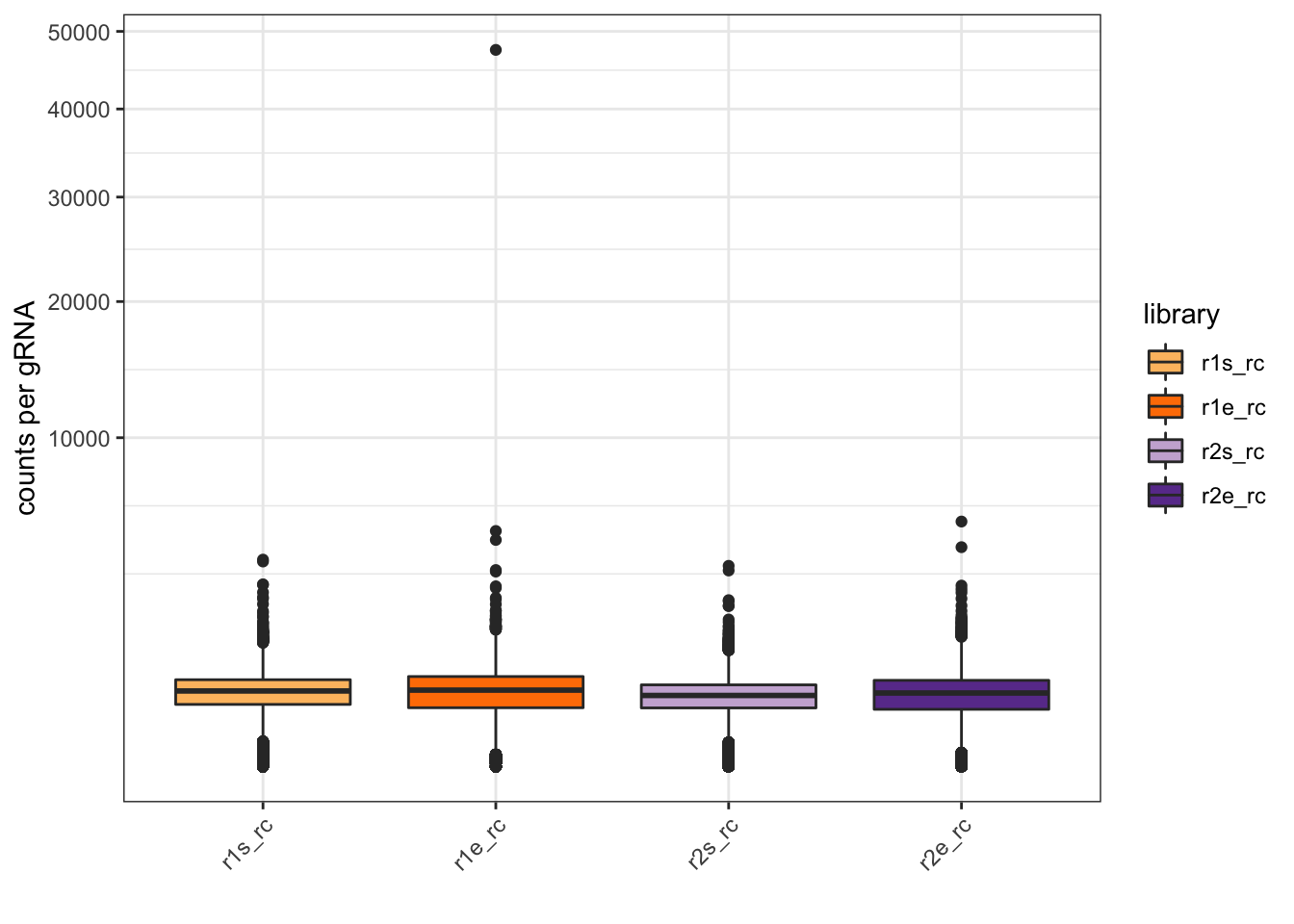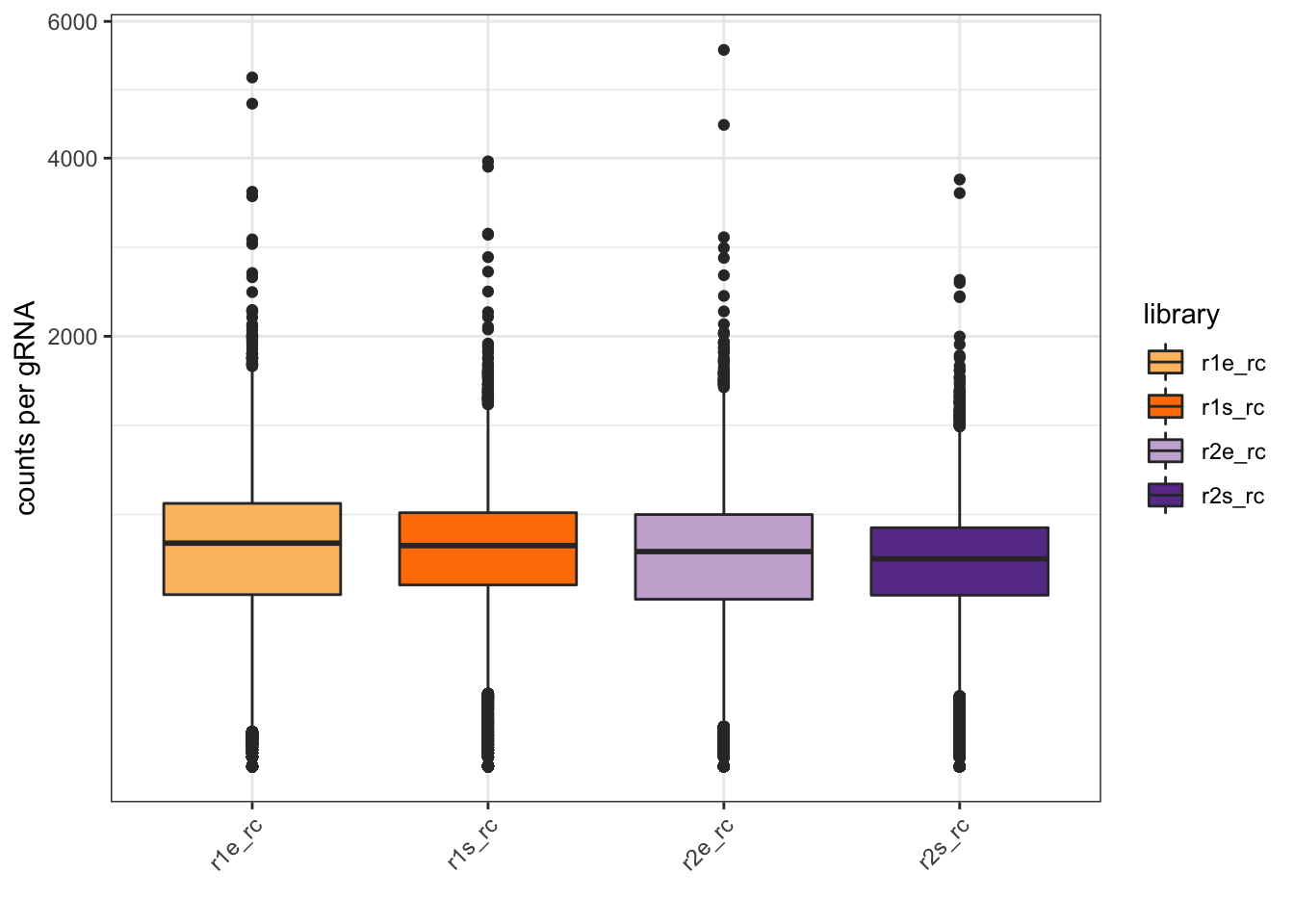# Data normalization

``````# load library file (after liftover, now hg38):

## note: spacer sequences were counted with count_spacers.py and the following script:
## ~/Sync/Analysis/CRISPR_scan/171230_CRISPRi-scan_count_data/Spacer_count_server.sh
colnames(r1s) <- "r1s_rc"
r1s\$r1s_norm <- (r1s[,2]+1)/sum(r1s[,2])

colnames(r1e) <- "r1e_rc"
r1e\$r1e_norm <- (r1e[,2]+1)/sum(r1e[,2])

colnames(r2s) <- "r2s_rc"
r2s\$r2s_norm <- (r2s[,2]+1)/sum(r2s[,2])

colnames(r2e) <- "r2e_rc"
r2e\$r2e_norm <- (r2e[,2]+1)/sum(r2e[,2])

# merge:
libm <- merge(lib,r1s,by.x = "protospacer.sequence",by.y = "V1")
libm <- merge(libm,r1e,by.x = "protospacer.sequence",by.y = "V1")
libm <- merge(libm,r2s,by.x = "protospacer.sequence",by.y = "V1")
libm <- merge(libm,r2e,by.x = "protospacer.sequence",by.y = "V1")

# calculate fold change rep1:
libm\$r1_fc <- libm\$r1e_norm/libm\$r1s_norm
libm\$r1_lfc <- log2(libm\$r1_fc)
# calculate fold change rep2:
libm\$r2_fc <- libm\$r2e_norm/libm\$r2s_norm
libm\$r2_lfc <- log2(libm\$r2_fc)

# Caveat:
# there are 5 more sequences in library than in count files
# this is due to the fact, that there are 5 sequences that are duplicated in library:
summary(r1s\$V1 %in% lib\$protospacer.sequence)``````
``````##    Mode    TRUE
## logical   26993``````
``summary(lib\$protospacer.sequence %in% r1s\$V1)``
``````##    Mode    TRUE
## logical   26998``````
``summary(duplicated(lib\$protospacer.sequence))``
``````##    Mode   FALSE    TRUE
## logical   26993       5``````
``````# these duplicates are gRNAs that work both as CRISPRn and CRISPRi controls:
lib[c(which(duplicated(lib\$protospacer.sequence)),which(duplicated(lib\$protospacer.sequence,fromLast = T))),]``````
``````##           gRNA.ID strand protospacer.sequence target        category chrom
## 26591   RFC3_ig-1   <NA> GCCGATACTTGTCCACCCAG   RFC3 CRISPRi control  <NA>
## 26592   RFC3_ig-2   <NA> GGGACGGCTGGACTATCACA   RFC3 CRISPRi control  <NA>
## 26621 RPL23A_ig-1   <NA> GCGCCGAAAGCGAAGAAGGA RPL23A CRISPRi control  <NA>
## 26932  CDC23_ig-4   <NA> GAGTACCTCCATGGTCCCGG  CDC23 CRISPRi control  <NA>
## 26997  HAUS5_ig-2   <NA> GGAGCTAGCGCAGGAAGCGC  HAUS5 CRISPRi control  <NA>
## 25752  CDC23_ng-1   <NA> GAGTACCTCCATGGTCCCGG  CDC23 CRISPRn control  <NA>
## 25842  HAUS5_ng-1   <NA> GGAGCTAGCGCAGGAAGCGC  HAUS5 CRISPRn control  <NA>
## 26022   RFC3_ng-1   <NA> GGGACGGCTGGACTATCACA   RFC3 CRISPRn control  <NA>
## 26024   RFC3_ng-3   <NA> GCCGATACTTGTCCACCCAG   RFC3 CRISPRn control  <NA>
## 26052 RPL23A_ng-1   <NA> GCGCCGAAAGCGAAGAAGGA RPL23A CRISPRn control  <NA>
##       chromStart chromEnd base_after_cut
## 26591         NA       NA             NA
## 26592         NA       NA             NA
## 26621         NA       NA             NA
## 26932         NA       NA             NA
## 26997         NA       NA             NA
## 25752         NA       NA             NA
## 25842         NA       NA             NA
## 26022         NA       NA             NA
## 26024         NA       NA             NA
## 26052         NA       NA             NA``````
``````# save library with normalized read counts
save(libm,file = "hg38_library_counts_norm.Rda")``````

# Removal of outlier counts

``````# plot raw read counts:
cols <- grep("rc",colnames(libm),value = T)
dfm <- gather(libm,cols,key = "library",value="count")
dfm\$library <- factor(dfm\$library,levels = c("r1s_rc","r1e_rc","r2s_rc","r2e_rc"))
p <- ggplot(dfm)
p+geom_boxplot(aes(x=library,y=count,fill=library))+scale_y_sqrt()+
labs(y="counts per gRNA",x="",fill="library")+theme_bw()+theme(axis.text.x = element_text(angle=45,hjust = 1))+
scale_fill_manual(values = cp.paired[c(7:10)])````````````ggsave("plot_counts_boxplot_incl_outlier_incl_low-count.pdf",width = 5,height = 4.5)

# from the boxplot above, it is apparent that there is one extreme outlier in r1e:
quantile(libm\$r1e_rc,probs = c(0,0.25,0.5,0.75,0.9,0.99,1))``````
``````##    0%   25%   50%   75%   90%   99%  100%
##     0   319   539   748   961  1395 47514``````
``libm %>% filter(r1e_rc>40000)``
``````##   protospacer.sequence     gRNA.ID strand target        category chrom
## 1 GACAGACGGATCGGCAGGGC INTS12_ig-1   <NA> INTS12 CRISPRi control  <NA>
##   chromStart chromEnd base_after_cut r1s_rc     r1s_norm r1e_rc
## 1         NA       NA             NA   2607 0.0001825892  47514
##      r1e_norm r2s_rc     r2s_norm r2e_rc     r2e_norm    r1_fc   r1_lfc
## 1 0.003204523   1375 0.0001088352   1135 8.352333e-05 17.55045 4.133436
##       r2_fc    r2_lfc
## 1 0.7674294 -0.381894``````
``````# delete this outlier:
libm <- libm %>% filter(!r1e_rc>40000)

# plots raw read counts after outlier removal:
dfm <- gather(libm,cols,key = "library",value="count")
p <- ggplot(dfm)
p+geom_boxplot(aes(x=library,y=count,fill=library))+scale_y_sqrt()+
labs(y="counts per gRNA",x="",fill="library")+theme_bw()+theme(axis.text.x = element_text(angle=45,hjust = 1))+
scale_fill_manual(values = cp.paired[c(7:10)])````````````ggsave("plot_counts_boxplot_excl_outlier_incl_low-count.pdf",width = 5,height = 4.5)

# write count summary data to table:
df.summary <- do.call(cbind,lapply(libm[,cols], summary))
pdf("table_count_summaries_excl_outlier_incl_low-count.pdf", height=11, width=8.5)
grid.table(df.summary)
dev.off()``````
``````## quartz_off_screen
##                 2``````

# Overview of low-count gRNAs

``````# overview of raw count data at start of screen:
quantile(libm\$r1s_rc,probs = c(0.01,0.02,0.05,0.1,0.2,0.25,0.5,0.75,1))``````
``````##   1%   2%   5%  10%  20%  25%  50%  75% 100%
##    1    7   61  162  309  356  527  696 3958``````
``quantile(libm\$r2s_rc,probs = c(0.01,0.02,0.05,0.1,0.2,0.25,0.5,0.75,1))``
``````##   1%   2%   5%  10%  20%  25%  50%  75% 100%
##    1    7   56  145  275  317  466  616 3724``````
``mean(c(libm\$r1s_rc,libm\$r2s_rc))``
``##  498.7146``
``````# overview of raw count data at end of screen:
quantile(libm\$r1e_rc,probs = c(0.01,0.02,0.05,0.1,0.2,0.25,0.5,0.75,1))``````
``````##   1%   2%   5%  10%  20%  25%  50%  75% 100%
##    0    5   38  108  259  319  539  748 5131``````
``quantile(libm\$r2e_rc,probs = c(0.01,0.02,0.05,0.1,0.2,0.25,0.5,0.75,1))``
``````##   1%   2%   5%  10%  20%  25%  50%  75% 100%
##    0    6   35  103  248  302  499  686 5550``````
``````# absent gRNAs:
summary(libm\$r1s_rc==0 | libm\$r1e_rc==0 | libm\$r2s_rc==0 | libm\$r2e_rct==0) # number of gRNAs that have count = 0 in any library``````
``````##    Mode
## logical``````
``summary(libm\$r1s_rc==0 & libm\$r1e_rc==0 & libm\$r2s_rc==0 & libm\$r2e_rct==0) # number of gRNAs that are not present in any library``
``````##    Mode
## logical``````
``summary(libm\$r1s_rc==0 & libm\$r1e_rc==0) # number of gRNAs not present in replicate 1``
``````##    Mode   FALSE    TRUE
## logical   26805     192``````
``summary(libm\$r2s_rc==0 & libm\$r2e_rc==0) # number of gRNAs not present in replicate 2``
``````##    Mode   FALSE    TRUE
## logical   26811     186``````
``````# very few gRNAs go from high counts to 0
summary(libm\$r1s_rc>50 & libm\$r1e_rc==0)``````
``````##    Mode   FALSE    TRUE
## logical   26995       2``````
``summary(libm\$rep2_start>50 & libm\$rep2_end==0)``
``````##    Mode
## logical``````
``````# but this depletion seems to be real as it is consistent between replicates:
libm[which((libm\$rep1_start>50 & libm\$rep1_end==0) | (libm\$rep2_start>50 & libm\$rep2_end==0)),]``````
``````##   protospacer.sequence gRNA.ID              strand
##   target               category             chrom
##   chromStart           chromEnd             base_after_cut
##  r1s_rc               r1s_norm             r1e_rc
##  r1e_norm             r2s_rc               r2s_norm
##  r2e_rc               r2e_norm             r1_fc
##  r1_lfc               r2_fc                r2_lfc
## <0 rows> (or 0-length row.names)``````
``````# calculate delta lfc between replicates to see at which raw read count variability starts to increase:
libm <- libm %>% mutate(delta_lfc=abs(r1_lfc-r2_lfc))

# plot delta lfc in relation to raw read count (starting libraries) with proposed cutoff:
dfm <- gather(libm,r1s_rc,r2s_rc,key = "library",value="raw_count")
ggplot(dfm)+geom_point(aes(x=raw_count,y=delta_lfc),alpha=0.5)+
geom_vline(xintercept = 50,color="red")+
facet_wrap(~library,ncol = 1)+
coord_cartesian(xlim = c(0,500))+theme_bw()``````